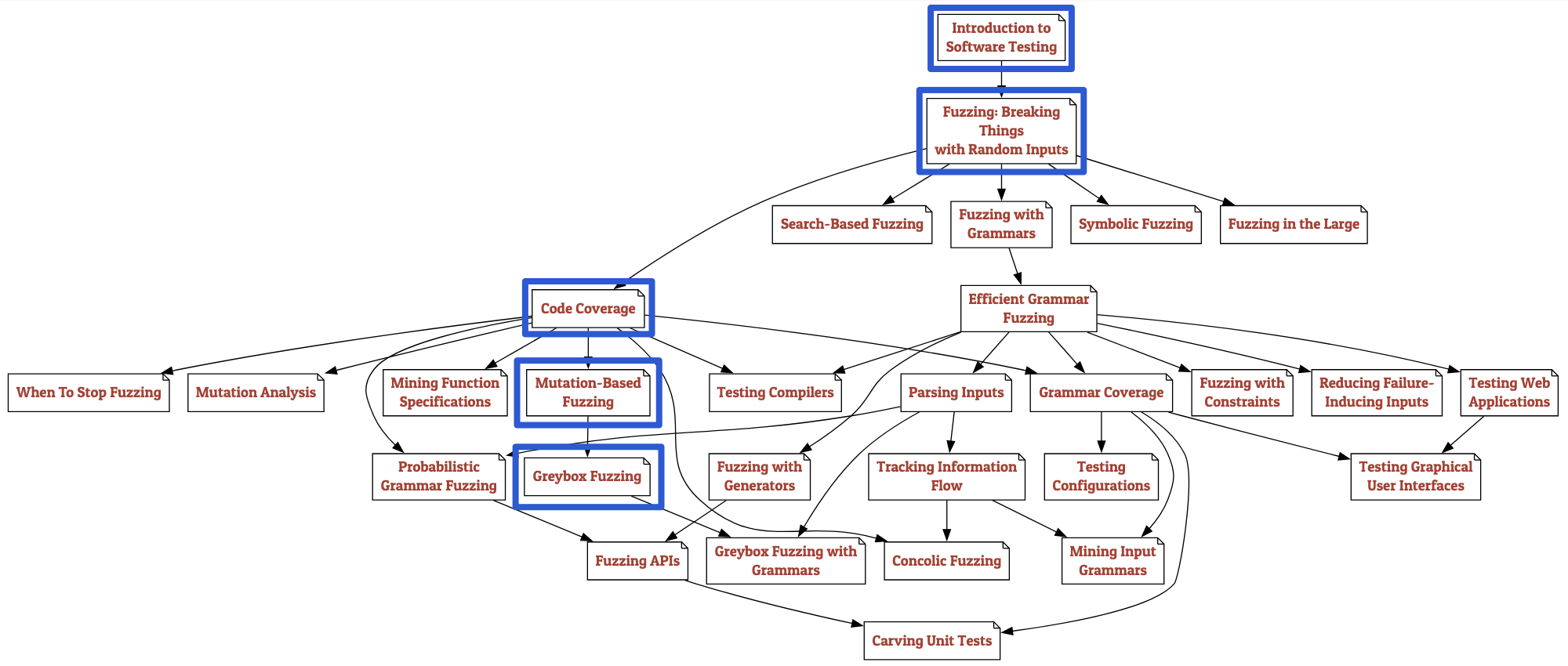## 1. 前言图1 - TFB学习路线及本笔记覆盖内容

1. Whitebox Fuzzing：在有目标程序的完整源代码或二进制代码的情况下进行，利用程序的结构信息来生成测试用例。其特点是更容易发现隐藏和深层的漏洞，但需要对代码或二进制进行深入分析，因此通常更为耗时。
2. Greybox Fuzzing：介于Whitebox和Blackbox之间，具有部分程序内部知识。它结合了Blackbox的速度和Whitebox的深度；通常使用轻量级的代码覆盖率信息来指导测试。
3. Blackbox Fuzzing：无需知道程序内部信息，只对程序的输入和输出进行测试，不依赖源代码或二进制分析。它速度更快，但可能错过一些深层次的漏洞。

1. Generation-based Fuzzing：即从零开始，根据某种预定义的规范或模型生成测试数据。
2. Mutation-based Fuzzing：从现有的合法输入数据开始，通过应用一系列随机或半随机变化来产生新的测试用例。

## 2. 从Flanker博客学到的…

Flanker曾经写过两篇关于Fuzzing的非常精彩的文章89，我将其中的要点总结如下：

Flanker第二篇则介绍了Fuzzing相关的二进制重写（rewriting）技术和动态追踪（dynamic tracing）技术。细节不再展开。这两篇文章内容文笔俱佳，高屋建瓴又能随时深入浅出，文字充满哲思和人文情怀，十分推荐阅读。

## 3. TFB：软件测试入门

### 3.1 `assert`断言

``````def my_sqrt(x):
approx = None
guess = x / 2
while approx != guess:
approx = guess
guess = (approx + x / approx) / 2
return approx

# >>> my_sqrt(2) * my_sqrt(2)
# 1.9999999999999996
``````

``````def assertEquals(x, y, epsilon=1e-8): assert abs(x - y) < epsilon
``````

### 3.2 `Timer`与`timeout`

TFB提供了`Timer`模块来帮助我们记录测试的运行时间：

``````from Timer import Timer

with Timer() as t:
for i in range(10000):
x = 1 + random.random() * 1000000
assertEquals(my_sqrt(x) * my_sqrt(x), x)
print(t.elapsed_time())

# 0.18018318619579077
``````

``````from ExpectError import ExpectTimeout

def sqrt_program(arg: str) -> None:  # type: ignore
x = int(arg)
print('The root of', x, 'is', my_sqrt(x))

with ExpectTimeout(1): sqrt_program("-1")

# Traceback (most recent call last):
# ...
#     raise TimeoutError()
# TimeoutError (expected)
``````

## 4. TFB：Fuzzing入门实践

### 4.1 随机字符串测试

``````def fuzzer(max_length: int = 100, char_start: int = 32, char_range: int = 32) -> str:
string_length = random.randrange(0, max_length + 1)
out = ""
for i in range(0, string_length):
out += chr(random.randrange(char_start, char_start + char_range))
return out

# >>> fuzzer(50, ord('A'), 26)
# 'FPRBKYKNRRVHHIZKAGSARFQFUUCEZJREMIGWBQMJGKCB'
``````

``````trials = 100
program = "bc"
runs = []

for i in range(trials):
data = fuzzer()
with open(FILE, "w") as f: f.write(data)
result = subprocess.run([program, FILE],
stdin=subprocess.DEVNULL,
stdout=subprocess.PIPE,
stderr=subprocess.PIPE,
universal_newlines=True)
runs.append((data, result))
``````

### 4.2 报告异常

``````def crash_if_too_long(s):
buffer = "Thursday"
if len(s) > len(buffer): raise ValueError
``````

TFB提供的`ExpectError`模块可以报告异常但同时确保流程继续执行：

``````from ExpectError import ExpectError

trials = 100
with ExpectError():
for i in range(trials):
s = fuzzer()
crash_if_too_long(s)

# Traceback (most recent call last):
#   File "/tmp/ipykernel_41/292568387.py", line 5, in <module>
#     crash_if_too_long(s)
#   File "/tmp/ipykernel_41/2784561514.py", line 4, in crash_if_too_long
#     raise ValueError
# ValueError (expected)
``````

### 4.3 检测与识别错误

``````clang -fsanitize=address -g -o program program.c
``````

### 4.4 Fuzzing基础架构

Runner类负责用给定输入运行被测程序，作为基类，它的实现非常简单：

``````class Runner:
# Test outcomes
PASS = "PASS"
FAIL = "FAIL"
UNRESOLVED = "UNRESOLVED"

def __init__(self) -> None: pass
def run(self, inp: str) -> Any: return (inp, Runner.UNRESOLVED)
``````

``````class ProgramRunner(Runner):
def __init__(self, program: Union[str, List[str]]) -> None:
self.program = program

def run_process(self, inp: str = "") -> subprocess.CompletedProcess:
return subprocess.run(self.program,
input=inp,
stdout=subprocess.PIPE,
stderr=subprocess.PIPE,
universal_newlines=True)

def run(self, inp: str = "") -> Tuple[subprocess.CompletedProcess, Outcome]:
result = self.run_process(inp)
if result.returncode == 0: outcome = self.PASS
elif result.returncode < 0: outcome = self.FAIL
else: outcome = self.UNRESOLVED
return (result, outcome)
``````

Fuzzer类负责生成测试数据（`fuzz`方法）及启动Runner进行测试（`run``runs`方法）：

``````class Fuzzer:
def __init__(self) -> None: pass
def fuzz(self) -> str: return ""

def run(self, runner: Runner = Runner()) \
-> Tuple[subprocess.CompletedProcess, Outcome]:
return runner.run(self.fuzz())

def runs(self, runner: Runner = PrintRunner(), trials: int = 10) \
-> List[Tuple[subprocess.CompletedProcess, Outcome]]:
return [self.run(runner) for i in range(trials)]
``````

``````class RandomFuzzer(Fuzzer):
def __init__(self, min_length: int = 10, max_length: int = 100,
char_start: int = 32, char_range: int = 32) -> None:
self.min_length = min_length
self.max_length = max_length
self.char_start = char_start
self.char_range = char_range

def fuzz(self) -> str:
string_length = random.randrange(self.min_length, self.max_length + 1)
out = ""
for i in range(0, string_length):
out += chr(random.randrange(self.char_start,
self.char_start + self.char_range))
return out
``````

## 5. TFB：Code Coverage

### 5.1 Python中的Coverage统计

``````def traceit(frame, event, arg):
if event == 'line':
global coverage
function_name = frame.f_code.co_name
lineno = frame.f_lineno
coverage.append(lineno)
return traceit
``````

``````coverage = []

def cgi_decode_traced(s: str) -> None:
global coverage
coverage = []
sys.settrace(traceit)  # Turn on
cgi_decode(s)
sys.settrace(None)    # Turn off

cgi_decode_trace("a+b")
``````

``````with Coverage() as cov: function_to_be_traced()
c = cov.coverage() # Tuple[str, int]
``````

`cov.coverage()`是一个集合，因此我们可以很方便地对不同的coverage做集合运算，从而进行coverage比较。

### 5.2 基础Fuzzing的Coverage

``````trials = 100

def population_coverage(population: List[str], function: Callable) \
-> Tuple[Set[Location], List[int]]:
cumulative_coverage: List[int] = []
all_coverage: Set[Location] = set()
for s in population:
with Coverage() as cov:
try: function(s)
except: pass
all_coverage |= cov.coverage()
cumulative_coverage.append(len(all_coverage))
return all_coverage, cumulative_coverage

def hundred_inputs() -> List[str]:
population = []
for i in range(trials): population.append(fuzzer())
return population

all_coverage, cumulative_coverage = \
population_coverage(hundred_inputs(), cgi_decode)

# all_coverage: {('cgi_decode', 9), ('cgi_decode', 12), ...}
# cumulative_coverage: [18, 19, 19, 19, ...]
``````

### 5.3 外部程序的Coverage统计

``````gcc --coverage -o cgi_decode cgi_decode.c
./cgi_decode 'my+email+is+rambo%40wohin.me'
gcov cgi_decode.c

# Lines executed:91.43% of 35
# Creating 'cgi_decode.c.gcov'
``````

### 5.4 Branch Coverage

``````def branch_coverage(trace):
coverage = set()
past_line = None
for line in trace:
if past_line is not None: coverage.add((past_line, line))
past_line = line
return coverage

# (('cgi_decode', 15), ('cgi_decode', 16)),
# (('cgi_decode', 16), ('cgi_decode', 17)),
# ...
``````

ChatGPT进一步解答了我的疑问：通过记录并拼接连续执行的两条语句，我们可以得知程序的执行流程如何在各个语句间转移。在一个包含分支结构（如`if-else``switch-case`）的程序中，根据不同的输入或状态，代码可能会走不同的分支。因此，跟踪连续执行的语句可以帮助我们了解哪些分支被执行了，哪些没有。